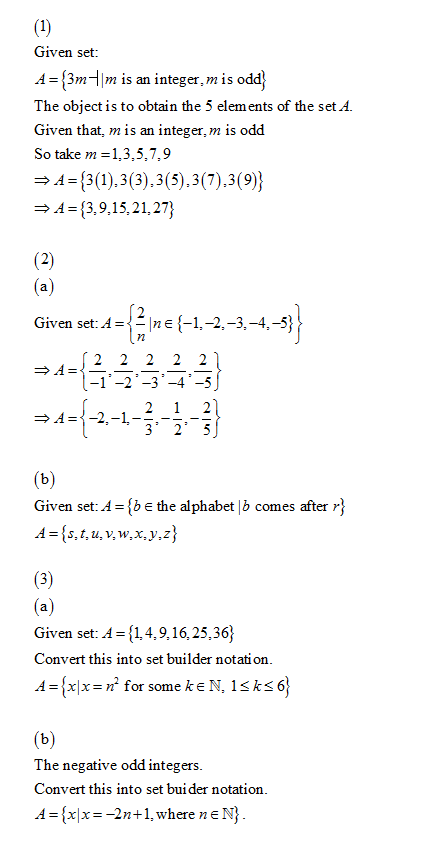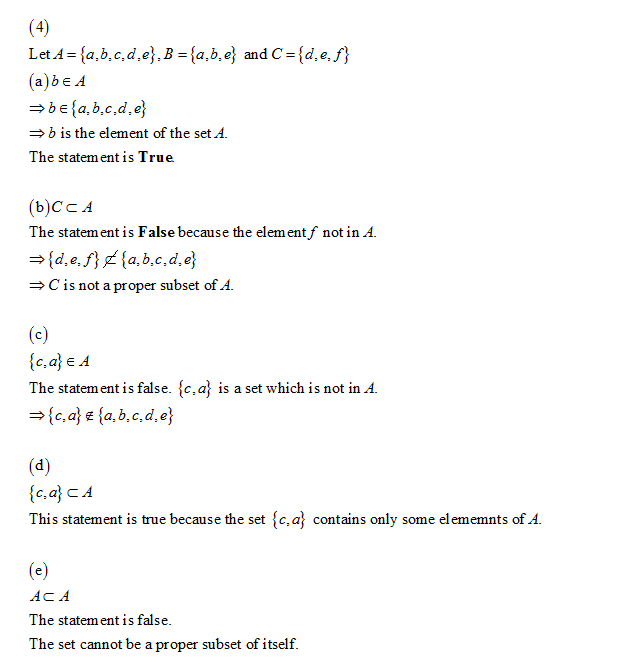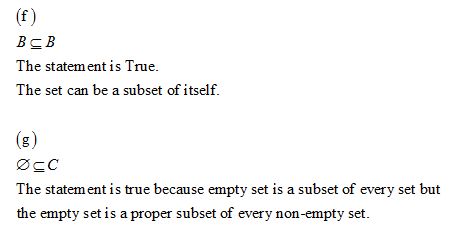1. List exactly five elements of the following set:

Don't use plagiarized sources. Get Your Custom Essay on
GET AN ESSAY WRITTEN FOR YOU FROM AS LOW AS \$13/PAGE

{3m ┤| m is an integer,m is odd}

2. List all elements of the following sets.

a. {2/n | n ∈{-1,-2,-3,-4,-5}}

b. {b ∈the alphabet | b comes after r}

3. Describe the following sets using set-builder notation.

a. {1, 4, 9, 16, 25, 36}

b. The negative odd integers

4. Let A = {a, b, c, d, e}, B = {a, b, e}, and C = {d, e, f}. Which of the following statements are true? Which are false? ⊂ means proper subset.

a. b ∈A

b. C ⊂A

c. {c,a}∈A

d. {c,a}⊂A

e. A ⊂A

f. B ⊆B

g. ∅ ⊆C

5. Let A, B, and C be as in #4 and let U = {a, b, c, d, e, f, g}. Determine:

a. A ∩B

b. A ∩C

c. A ∪B

d. A ∪C

e. A-B

f. A-C

g. B-A

h. C-A

i. A^C

j. B ⨁▒C

6. Given that U = all students at College, I represents all IT students, P represents all part-time students, and F represents all students on financial aid, draw Venn diagrams illustrating this situation and shade in the following sets: a. Part-time IT students

b. Non-IT full-time students on financial aid

7. Let |U| = 70,000, |I| = 1400, |P| = 48,000 and |F| = 51,000. Also assume that the number of part-time IT students is 700; 400 of which are on financial aid, and that there are 950 IT students on financial aid. Determine the number of students who are:

a. Full-time IT students

b. Full-time IT students who are not on financial aid

8. Let A, B and C be as in #4 and let U = {a, b, c, d, e, f}. List the elements of:

a. B × C

b. C × C^C

9. List all 3-element sets in the power set of {1, 2, 3, 4, 5}.

10. Find the binary representation of 493. (Must show all work)

11. What positive integer has this binary representation: 110110101? (must show all work)

12. Calculate the following sum (show all work):

∑_(k=1)^3▒(k^3+k)